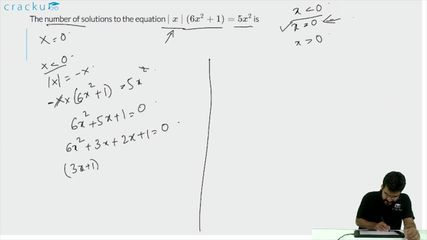Question 99

# The number of solutions to the equation $$\mid x \mid (6x^2 + 1) = 5x^2$$ is

Solution

For x <0, -x($$6x^2+1$$) = $$5x^2$$

=> ($$6x^2+1$$) = -5x

=> ($$6x^2 + 5x+ 1$$) = 0

=>($$6x^2 + 3x+2x+ 1$$) = 0

=> (3x+1)(2x+1)=0    =>x=$$\ -\frac{\ 1}{3}$$  or x=$$\ -\frac{\ 1}{2}$$

For x=0, LHS=RHS=0    (Hence, 1 solution)

For x >0, x($$6x^2+1$$) = $$5x^2$$

=> ($$6x^2 - 5x+ 1$$) = 0

=>(3x-1)(2x-1)=0    =>x=$$\ \frac{\ 1}{3}$$   or   x=$$\ \frac{\ 1}{2}$$

Hence, the total number of solutions = 5

### View Video Solution